# Reference Evapotranspiration (ETref)

Reference crop evapotranspiration or reference evapotranspiration, denoted as ETo or ETref, is the estimation of the evapotranspiration from the "reference surface." The reference surface is a hypothetical grass reference crop with an assumed crop height of 0.12 m, a fixed surface resistance of 70 s/m and an albedo of 0.23. The reference surface closely resembles an extensive surface of green, well-watered grass of uniform height, actively growing and completely shading the ground. The fixed surface resistance of 70 s/m implies a moderately dry soil surface resulting from about a weekly irrigation frequency.

For ETref, you have two options:

1. Enter ETref directly (some climate stations provide derived ETref as data), OR

2. Calculate it using the Penman-Monteith equation.  This approach has its own data requirements, with various options.  Penman-Monteith requires:

1. Minimum and maximum daily temperature.

2. Relative humidity.  Depending on the availability of data, different equations are used.  The following are the data requirements, in decreasing order of preference.

1. Minimum and maximum daily relative humidity, OR

2. Maximum daily relative humidity, OR

3. Average daily relative humidity, OR

4. If humidity data is not available, and estimate can be obtained by assuming that the dew point temperature is the same as the daily minimum temperature.

3. Solar radiation.  Depending on the availability of data, different equations are used.  The following are the data requirements, in decreasing order of preference.

1. Enter solar radiation data directly, OR

2. Hours of sunshine per day, OR

3. Cloudiness fraction, OR

4. If neither sunshine hours nor cloudiness fraction are available, solar radiation can be estimated using the Hargreaves formula, based on minimum and maximum daily temperature and an adjustment coefficient (Krs)

4. Wind speed.  (An adjustment can be made if the wind speed measurement height is known.)

5. Latitude and altitude of the climate measurement station.

Depending on the setting in General: Basic Parameters, the values for climate data can either be entered once for each catchment and will apply to all the land use branches within that catchment, or they will be entered separately for each branch within each catchment. This second option might be necessary if there is a large variation in the elevation among different land uses within a catchment. Alternatively, the catchment could be divided into several different catchment nodes according to elevation, so that the climate within each catchment did not vary by land use.

The calculation methods implemented in the MABIA Method are those of the FAO Penman-Monteith equation as outlined in the FAO Irrigation and Drainage Paper 56. This equation can be written as follows: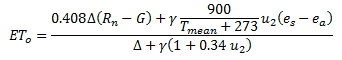where

ETo = reference evapotranspiration [mm/day],
Rn = net radiation at the crop surface [MJ/m^2/day],
G = soil heat flux density [MJ/m^2/day] which can be neglected (G=0),
Tmean = mean air temperature [°C],
u2 = wind speed measured at 2 m height [m/s],
es = saturation vapor pressure [kPa],
ea = actual vapor pressure [kPa],
es-ea = saturation vapor pressure deficit [kPa],
D
= slope vapor pressure curve [kPa/°C],
g
= psychrometric constant [kPa/°C].

The net radiation, Rn, is the difference between the incoming net shortwave radiation (Rns  ) and the outgoing net long wave radiation (Rnl):

Rn  = Rns  – Rnl

## Net solar or net shortwave radiation

The net shortwave radiation resulting from the balance between incoming and reflected solar radiation is given by:

Rns  = (1-D)  Rs

where

Rns = net shortwave radiation [MJ/m^2/day],
D
= albedo or canopy reflection coefficient for the reference crop [dimensionless] (a fixed value of 0.23 is used),
R

The net long wave radiation, Rnl, is given by: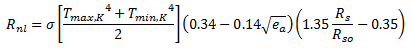where

Rnl = net long wave radiation [MJ/m^2/day],
s
= Stefan-Boltzmann constant [ 4.903 10^-9 MJ/K^4/m^2/day],
T
max = K maximum absolute temperature during the 24-hour period [K = °C + 273.16],
T
min = K minimum absolute temperature during the 24-hour period [K = °C + 273.16],
e
a = actual vapor pressure [kPa],
R
s/Rso = relative shortwave radiation (limited to <= 1.0)
R
R

If the solar radiation, Rs, is not measured, it can be calculated with the Angstrom formula, which relates solar radiation to extraterrestrial radiation and relative sunshine duration: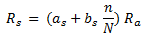where

Rs = solar or shortwave radiation [MJ/m^2/day],
n = actual duration of sunshine [hour],
N = maximum possible duration of sunshine or daylight hours [hour],
n/N = relative sunshine duration [fraction],
as = regression constant, expressing the fraction of extraterrestrial radiation reaching the earth on overcast days (n = 0),
as+bs= fraction of extraterrestrial radiation reaching the earth on clear days   (n = N).

The default values for as and bs are 0.25 and 0.50.

An alternative to entering the actual duration of sunshine (n) is to enter the cloudiness fraction, which is used in place of n/N.  When neither n nor cloudiness fraction is available, Rs can be estimated using the Hargreaves formula: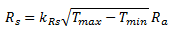where

Tmax = maximum air temperature [°C],
Tmin = minimum air temperature [°C],
kRs = adjustment coefficient (between 0.16 and 0.19) [°C-0.5].

The calculation of the clear-sky radiation, Rso, when n = N, is required for computing net long wave radiation.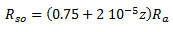where

z = station elevation above sea level [m],

The extraterrestrial radiation, Ra, for each day of the year and for different latitudes is estimated from the solar constant, the solar declination and the time of the year by:where

Gsc = solar constant = 0.0820 MJ/m^2/min,
dr = inverse relative distance Earth-Sun,
ws = sunset hour angle [rad],

The latitude, φ, expressed in radians is positive for the northern hemisphere and negative for the southern hemisphere. The conversion from decimal degrees to radians is given by: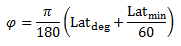The inverse relative distance Earth-Sun, dr, and the solar declination, δ, are given by: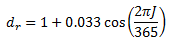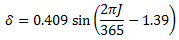where

J = number of the day in the year between 1 (1 January) and 365 or 366 (31 December).

The sunset hour angle, ws, is given by:

ws  = arccos (-tan(φ) tan(δ) )

## Daylight hours

The daylight hours, N, are given by:

N = 24 * ws/p

## Psychrometric constant

The psychrometric constant, g, is given by: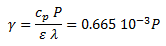where

g = psychrometric constant [kPa/°C],
P = atmospheric pressure [kPa],
l
= latent heat of vaporization = 2.45 [MJ/kg],
cp = specific heat at constant pressure = 1.013 10-3 [MJ/kg/°C],
e
= ratio molecular weight of water vapor/dry air = 0.622.

The value of the latent heat varies as a function of temperature. However, because λ varies only slightly over normal temperature ranges, a single value of 2.45 MJ/kg is used. (This value corresponds to an air temperature of about 20°C.)

## Atmospheric pressure

The atmospheric pressure, P, is the pressure exerted by the weight of the earth's atmosphere: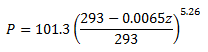where

P = atmospheric pressure [kPa],
z = elevation above sea level [m]

## Mean Daily air temperature

Tmean = (Tmax + Tmin) / 2

where

Tmean mean daily air temperature [°C],
T
min = minimum daily air temperature [°C],
T
max = maximum daily air temperature [°C].

## Wind speed

For the calculation of evapotranspiration, wind speed measured at 2 m above the surface is required. To adjust wind speed data obtained from instruments placed at elevations other than the standard height of 2 m, a logarithmic wind speed profile may be used for measurements above a short grassed surface: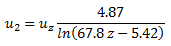where

u2 = wind speed at 2 m above ground surface [m/s],
uz = measured wind speed at z m above ground surface [m/s],
z = height of measurement above ground surface [m].

## Mean saturation vapor pressure

The mean saturation vapor pressure is the mean of the saturation vapor pressures at maximum and minimum air temperatures for the day:where

es = saturation vapor pressure [kPa],
e
°(Tmax) = saturation vapor pressure at the mean daily maximum air temperature [kPa],
e
°(Tmin) = saturation vapor pressure at the mean daily minimum air temperature [kPa].

## Saturation vapor pressure

The saturation vapor pressure, eo, is a function of air temperature:

eo (T) = 0.6108 exp ((17.27 T) / (T+237.3))

where

eo(T) = saturation vapor pressure at the air temperature T [kPa],
T = air temperature [°C].

## Actual vapor pressure

The actual vapor pressure, ea can also be calculated from the relative humidity. Depending on the availability of the humidity data, different equations are used:

1. Using RHmax and RHmin:

2.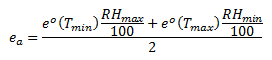3. Using RHmax :

4.5. Using RHmean:

6. In the absence of RHmax and RHmin, RHmean can be used to estimate ea:where RHmean is the mean relative humidity, defined as the average between RHmax and RHmin.

7. If Humidity data is not available

8. An estimate of actual vapor pressure, ea, can be obtained by assuming that dew point temperature (Tdew) is near the daily minimum temperature (Tmin) then,where

ea = actual vapor pressure [kPa],
e°(T
min) = saturation vapor pressure at daily minimum temperature [kPa],
e°(T
max) = saturation vapor pressure at daily maximum temperature [kPa],
RH
max = maximum relative humidity [%],
RH
min = minimum relative humidity [%].

## Slope vapor pressure curve

For the calculation of evapotranspiration by mean of various methods, the slope of the relationship between saturation vapor pressure and temperature, D, is required. The slope of the curve at a given temperature is given by: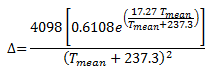where

D = slope of saturation vapor pressure curve at air temperature T [kPa/°C],

Tmean = mean air temperature [°C].